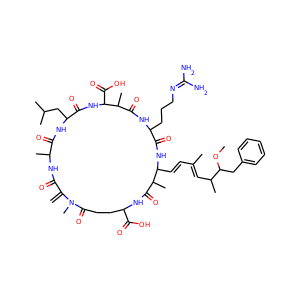877-202-0205     support@chemchart.com     @chemchartMicrocystin-LR
Weight 995.189 g/mol C49H74N10O12 11 10 1 15

# Microcystin-LR (101043-37-2)Score: #71 in Biochemistry , #327 in Biology , #343 in Chemistry
"What do you need help with?"### Alternate Names

• SMILES
CC1C(NC(=O)C(NC(=O)C(C(NC(=O)C(NC(=O)C(NC(=O)C(=C)N(C(=O)CCC(NC1=O)C(=O)O)C)C)CC(C)C)C(=O)O)C)CCCN=C(N)N)C=CC(=CC(C)C(CC2=CC=CC=C2)OC)C
• InChIKey
ZYZCGGRZINLQBL-UHFFFAOYSA-N